# DS 020 Chapter 3 Regular Languages and Regular

• Slides: 24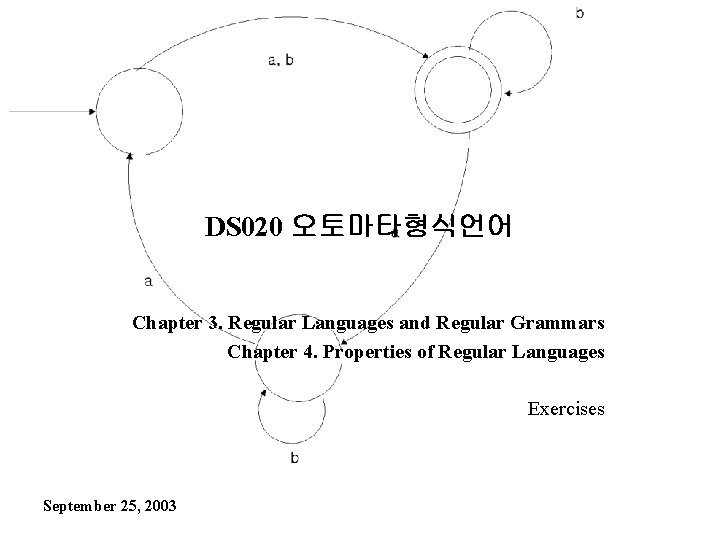DS 020 오토마타형식언어 Chapter 3. Regular Languages and Regular Grammars Chapter 4. Properties of Regular Languages Exercises September 25, 2003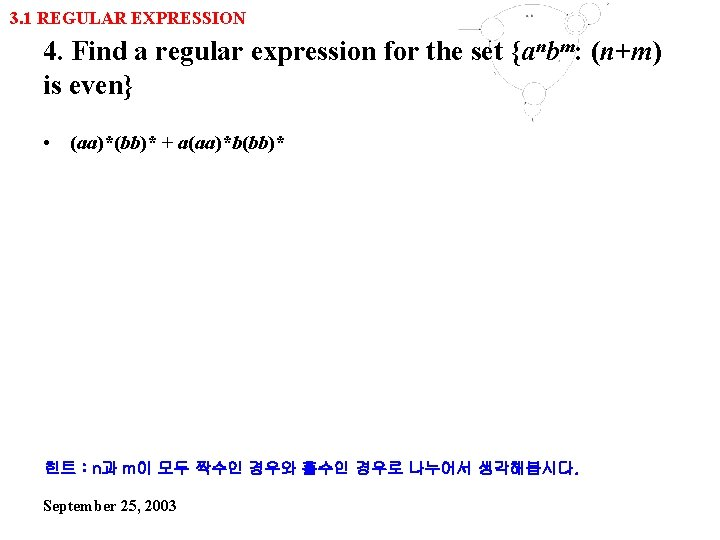3. 1 REGULAR EXPRESSION 4. Find a regular expression for the set {anbm: (n+m) is even} • (aa)*(bb)* + a(aa)*b(bb)* 힌트 : n과 m이 모두 짝수인 경우와 홀수인 경우로 나누어서 생각해봅시다. September 25, 2003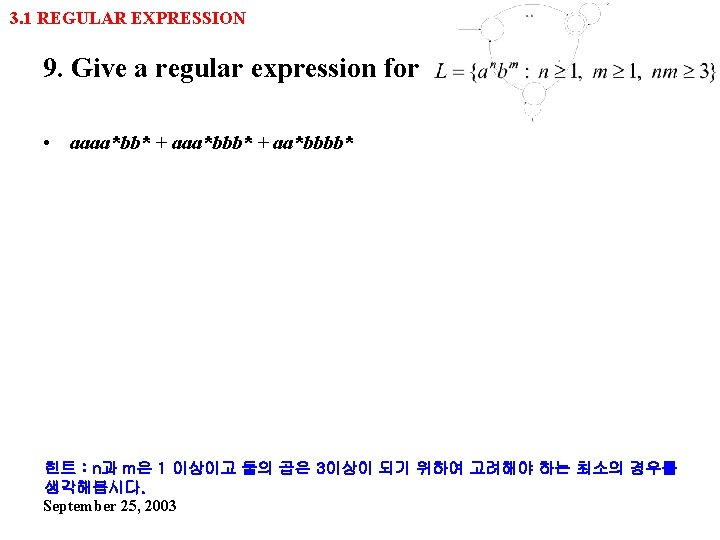3. 1 REGULAR EXPRESSION 9. Give a regular expression for • aaaa*bb* + aaa*bbb* + aa*bbbb* 힌트 : n과 m은 1 이상이고 둘의 곱은 3이상이 되기 위하여 고려해야 하는 최소의 경우를 생각해봅시다. September 25, 2003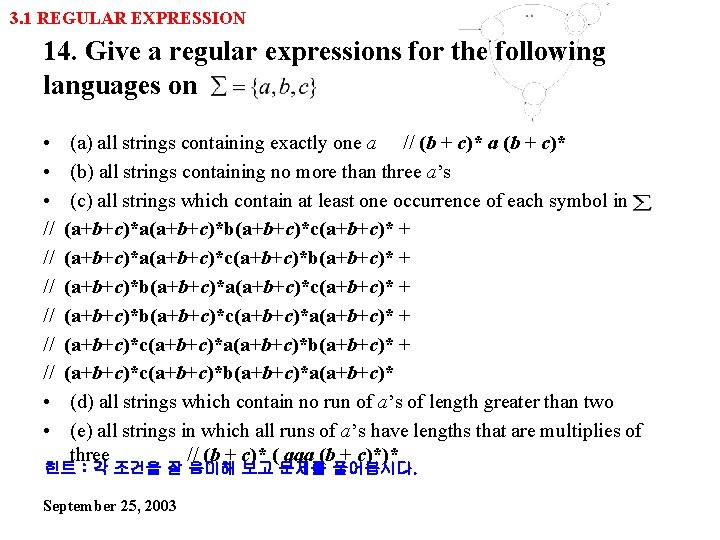3. 1 REGULAR EXPRESSION 14. Give a regular expressions for the following languages on • • • // // // • • (a) all strings containing exactly one a // (b + c)* a (b + c)* (b) all strings containing no more than three a’s (c) all strings which contain at least one occurrence of each symbol in (a+b+c)*a(a+b+c)*b(a+b+c)*c(a+b+c)* + (a+b+c)*a(a+b+c)*c(a+b+c)*b(a+b+c)* + (a+b+c)*b(a+b+c)*a(a+b+c)*c(a+b+c)* + (a+b+c)*b(a+b+c)*c(a+b+c)*a(a+b+c)* + (a+b+c)*c(a+b+c)*a(a+b+c)*b(a+b+c)* + (a+b+c)*c(a+b+c)*b(a+b+c)*a(a+b+c)* (d) all strings which contain no run of a’s of length greater than two (e) all strings in which all runs of a’s have lengths that are multiplies of three // (b + c)* ( aaa (b + c)*)* 힌트 : 각 조건을 잘 음미해 보고 문제를 풀어봅시다. September 25, 20033. 1 REGULAR EXPRESSION 22. Formal languages can be used to describe a variety of twodimensional figures. Chain-code languages are defined on the alphabet , where these symbols stand for unitlength straight lines in the directions up, down, right, and left, respectively. An example of this notation is urdl, which stands for the square with sides of init length. Draw pictures of the figures denoted by the expressions (rd)*, (urddru)*, and (ruldr)*. 힌트 : 직접 한번씩 그려봅시다. 간단한 모양이 반복되는군요. ^^ September 25, 2003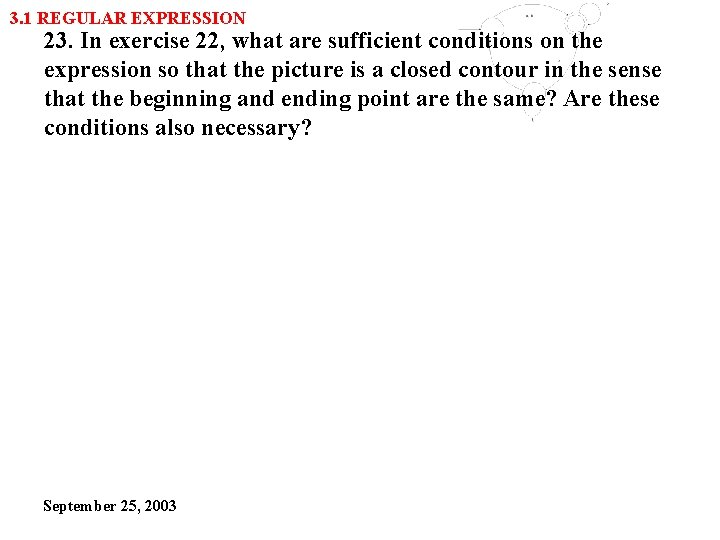3. 1 REGULAR EXPRESSION 23. In exercise 22, what are sufficient conditions on the expression so that the picture is a closed contour in the sense that the beginning and ending point are the same? Are these conditions also necessary? September 25, 2003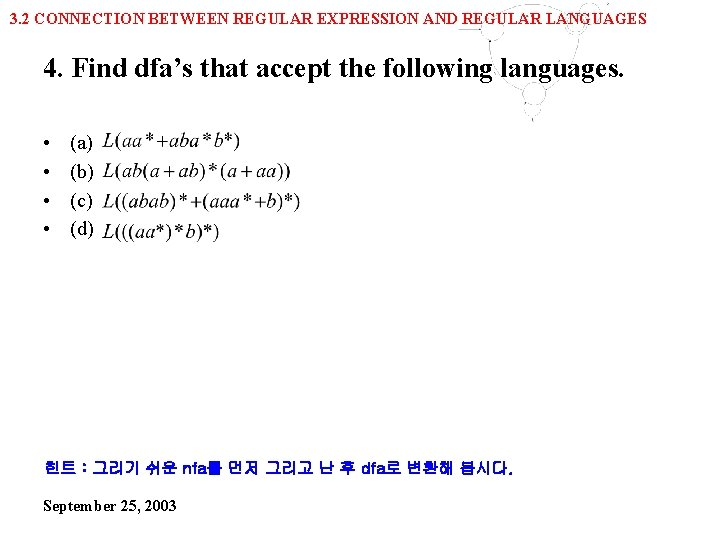3. 2 CONNECTION BETWEEN REGULAR EXPRESSION AND REGULAR LANGUAGES 4. Find dfa’s that accept the following languages. • • (a) (b) (c) (d) 힌트 : 그리기 쉬운 nfa를 먼저 그리고 난 후 dfa로 변환해 봅시다. September 25, 20033. 2 CONNECTION BETWEEN REGULAR EXPRESSION AND REGULAR LANGUAGES 10. Find regular expressions for the languages accepted by the following automata. b*aa*ab*a 힌트 : (a) one-way 문제는 그냥 쭉 따라가기만 하면 됩니다. 힌트 : (b) 초기 상태를 non-final으로 만든 후 state수를 줄여나가 봅시다. 힌트 : (c) final state의 수를 하나로 줄여봅시다. September 25, 20033. 2 CONNECTION BETWEEN REGULAR EXPRESSION AND REGULAR LANGUAGES 17. In some applications, such as programs that check spelling, we may not need an exact match of the pattern, only an approximate one, once the notion of an approximate match has been made precise, automata theory can be applied to construct approximate pattern matchers. As an illustration of this, consider patterns derived from the original ones by insertion of one symbol. Let L be a regular language on insert(L) = and define. In effect, insert(L) contains all the words created from L by inserting a spurious anywhere in a word. • (a) Given an NFA for L, show one can construct an NFA for insert(L) • (b) discuss how you might use this to write a pattern-recognition program for insert(L), using as input a regular expression for L. September 25, 2003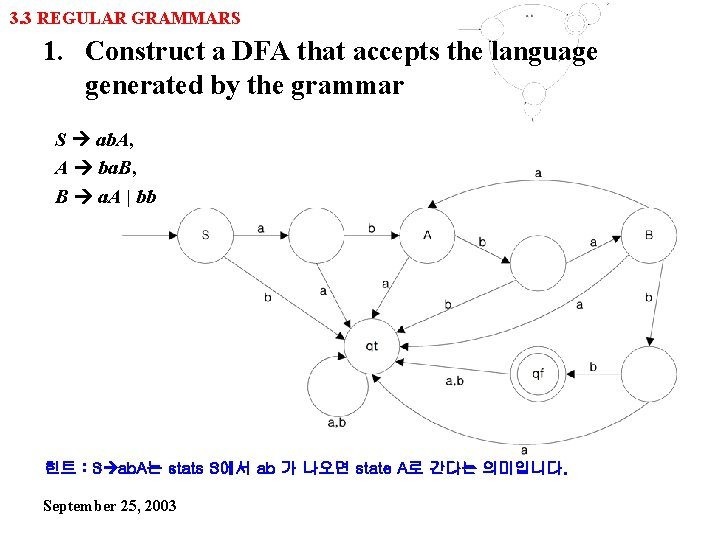3. 3 REGULAR GRAMMARS 1. Construct a DFA that accepts the language generated by the grammar S ab. A, A ba. B, B a. A | bb 힌트 : S ab. A는 stats S에서 ab 가 나오면 state A로 간다는 의미입니다. September 25, 2003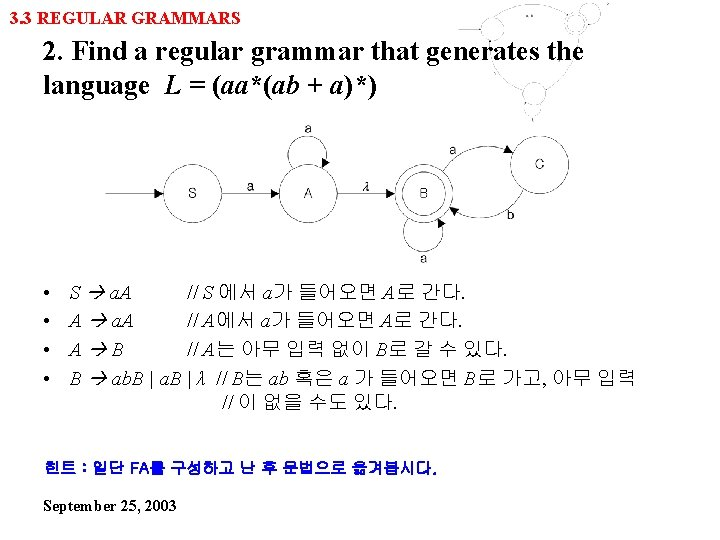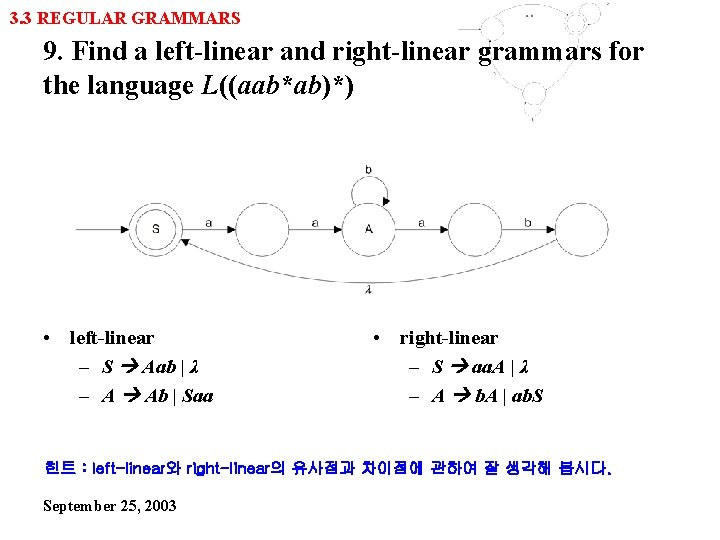3. 3 REGULAR GRAMMARS 9. Find a left-linear and right-linear grammars for the language L((aab*ab)*) • left-linear – S Aab | λ – A Ab | Saa • right-linear – S aa. A | λ – A b. A | ab. S 힌트 : left-linear와 right-linear의 유사점과 차이점에 관하여 잘 생각해 봅시다. September 25, 2003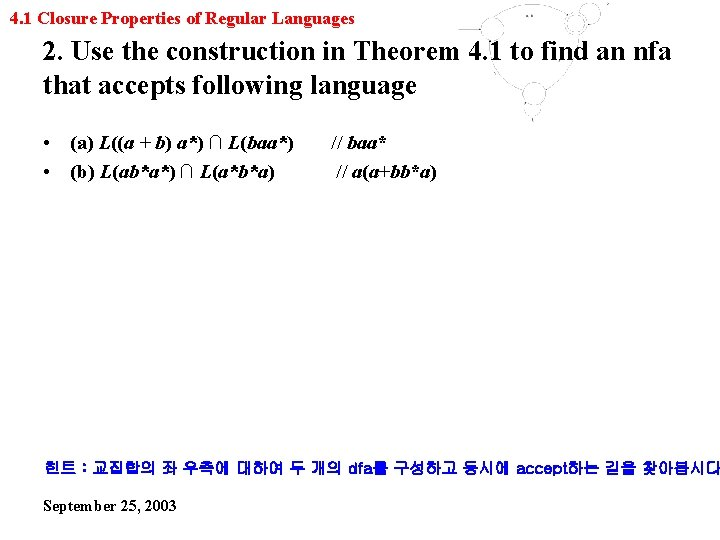4. 1 Closure Properties of Regular Languages 2. Use the construction in Theorem 4. 1 to find an nfa that accepts following language • (a) L((a + b) a*) ∩ L(baa*) • (b) L(ab*a*) ∩ L(a*b*a) // baa* // a(a+bb*a) 힌트 : 교집합의 좌 우측에 대하여 두 개의 dfa를 구성하고 동시에 accept하는 길을 찾아봅시다 September 25, 20034. 1 Closure Properties of Regular Languages 6. The symmetric difference of two sets S 1 and S 2 is defined as follow: Show that the family of regular languages is closed under symmetric difference • S 1ӨS 2={x: x∈S 1 or x∈S 2, but x is not in both S 1 and S 2} September 25, 20034. 1 Closure Properties of Regular Languages 10. Let L 1=L(a*baa*) and L 2=L(aba*). Find L 1/L 2 • a* 힌트 : 예제 4. 5를 그대로 따라서 해봅시다. ^^ September 25, 20034. 1 Closure Properties of Regular Languages 15. The left quotient of a language L 1 with respect to L 2 is defined as follow: Show that the family of regular languages is closed under left quotient with a regular language • L 2/L 1={y: x∈L 2, xy∈L 1} 힌트 : left quotient를 이해한 후 right quotient를 이해해 봅시다. September 25, 2003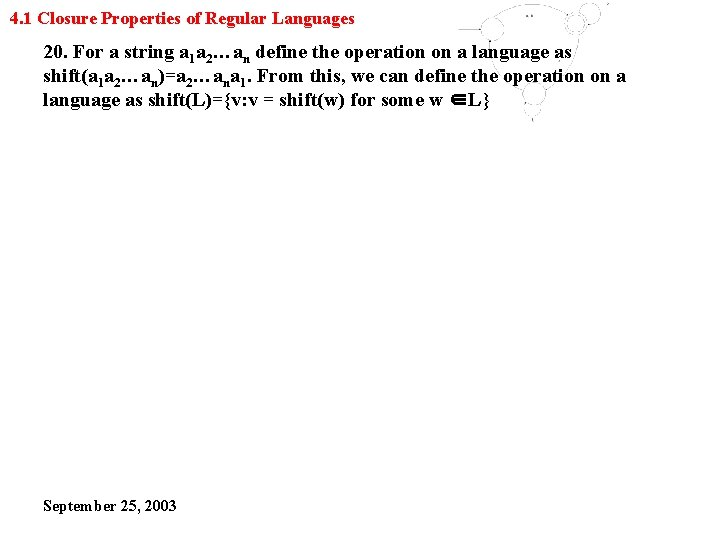4. 1 Closure Properties of Regular Languages 20. For a string a 1 a 2…an define the operation on a language as shift(a 1 a 2…an)=a 2…ana 1. From this, we can define the operation on a language as shift(L)={v: v = shift(w) for some w ∈L} September 25, 2003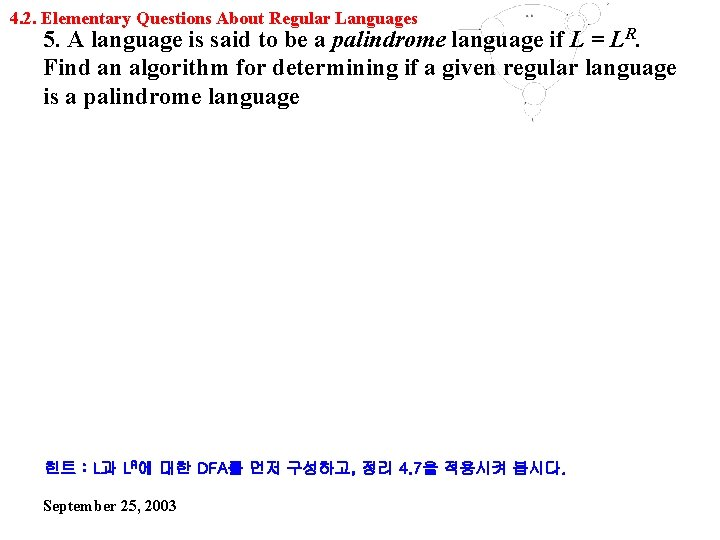4. 2. Elementary Questions About Regular Languages 5. A language is said to be a palindrome language if L = LR. Find an algorithm for determining if a given regular language is a palindrome language 힌트 : L과 LR에 대한 DFA를 먼저 구성하고, 정리 4. 7을 적용시켜 봅시다. September 25, 20034. 2. Elementary Questions About Regular Languages 9. Let L be a regular language on ∑ and w be any string in ∑*. Find an algorithm to determine if L contains any w such that is a substring of it, that is, such that, with u, v ∈∑* September 25, 2003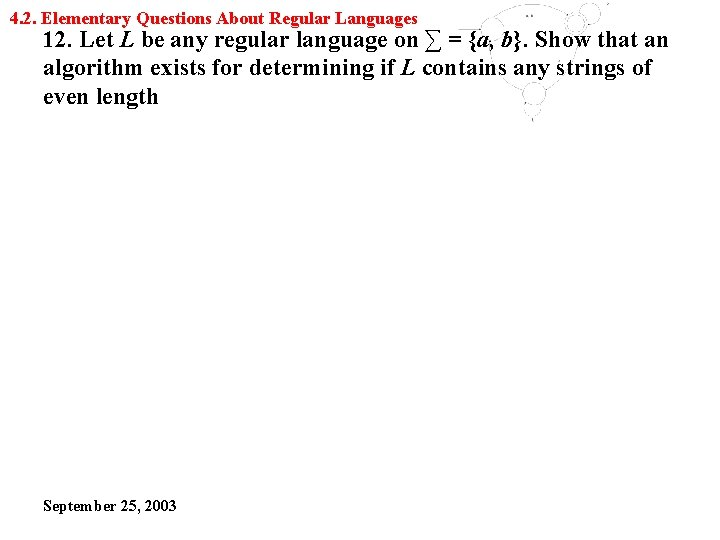4. 2. Elementary Questions About Regular Languages 12. Let L be any regular language on ∑ = {a, b}. Show that an algorithm exists for determining if L contains any strings of even length September 25, 2003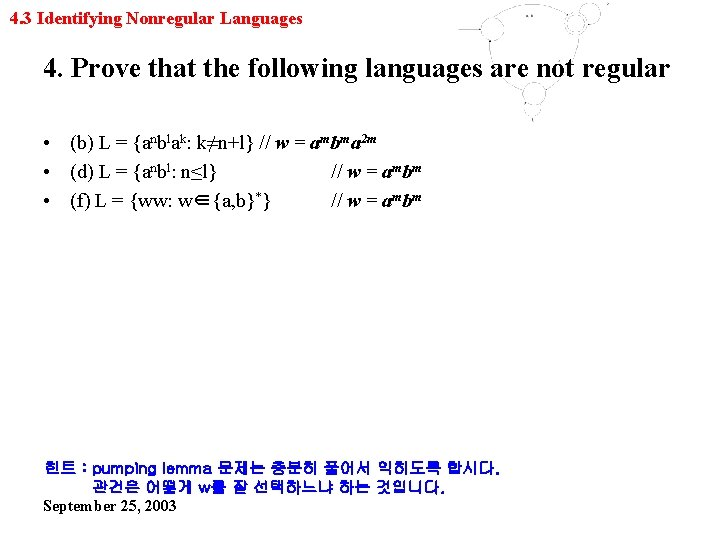4. 3 Identifying Nonregular Languages 4. Prove that the following languages are not regular • (b) L = {anblak: k≠n+l} // w = ambma 2 m • (d) L = {anbl: n≤l} // w = ambm • (f) L = {ww: w∈{a, b}*} // w = ambm 힌트 : pumping lemma 문제는 충분히 풀어서 익히도록 합시다. 관건은 어떻게 w를 잘 선택하느냐 하는 것입니다. September 25, 2003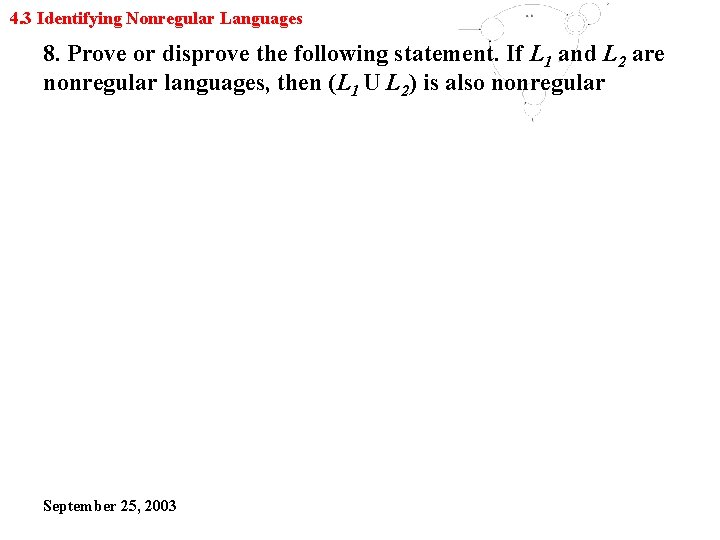4. 3 Identifying Nonregular Languages 8. Prove or disprove the following statement. If L 1 and L 2 are nonregular languages, then (L 1 U L 2) is also nonregular September 25, 2003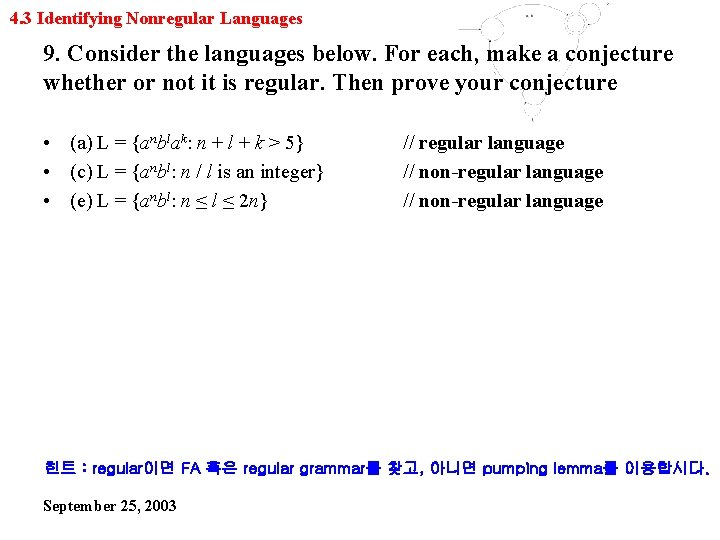4. 3 Identifying Nonregular Languages 9. Consider the languages below. For each, make a conjecture whether or not it is regular. Then prove your conjecture • (a) L = {anblak: n + l + k > 5} • (c) L = {anbl: n / l is an integer} • (e) L = {anbl: n ≤ l ≤ 2 n} // regular language // non-regular language 힌트 : regular이면 FA 혹은 regular grammar를 찾고, 아니면 pumping lemma를 이용합시다. September 25, 20034. 3 Identifying Nonregular Languages 15. Let P be an infinite but countable set, and associate with each p∈P a language Lp. The smallest set containing every Lp is the union over the infinite set P; it will be denoted by U p∈P Lp. Show by example that the family of regular languages is not closed under infinite union September 25, 2003